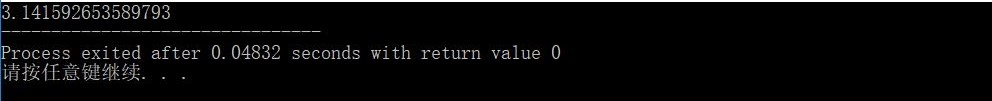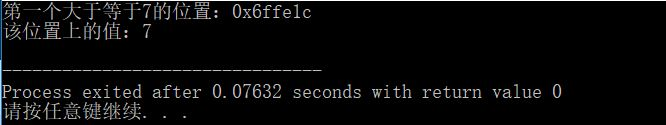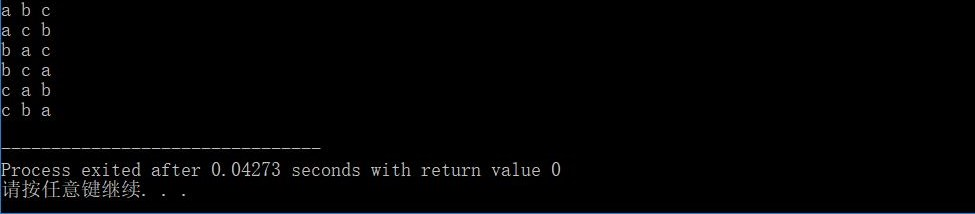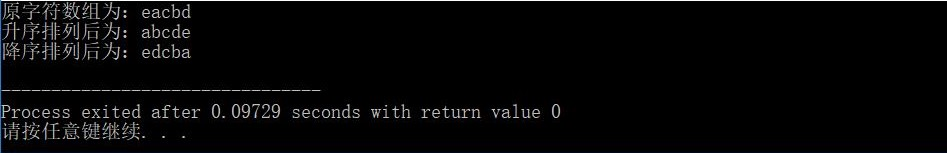# C/C++常用函数合集

C标准库

<string.h>头文件

1.memset()

2.memcpy()

3.strcpy()

4.strncpy()

5.stract()

6.getline()

7.strlen()

<ctype.h>头文件

1.tolower()/toupper()

2.isalpha（），isdigit（），isprint（）

<math.h>头文件

1.pow()

2.floor()

3.atan()

STL

<algorithm>头文件

1.min(),max()函数

2.lower_bound()

3.upper_bound（）

4.next_permutation()/prev_permutation()

6.Sort()

# <string.h>头文件

## 1.memset()

2.一般不用于初始化数字数组

#include<stdio.h>
#include<string.h>

int main(){
char str={'1','2','3','4','5'};

printf("原始字符数组:");
for(int i=0;i<5;i++)
printf("%c ",str[i]);
printf("\n");

//	内存初始化
memset(str,'\0',5);

printf("初始化后的字符数组:");
for(int i=0;i<5;i++)
printf("%c ",str[i]);

return 0;
}

## 2.memcpy()

char a,b;
memcpy(b,a,sizeof(b)); //将a的sizeof(b)个元素赋给b

## 3.strcpy()

char a,b;
strcpy(a,b);

## 4.strncpy()

2.如果src的长度小于n个字节，则以NULL填充dest直到复制完n个字节

## 6.getline()

 is 进行读入操作的输入流，常用cin str 已声明的用于存储读入内容的对象，常为string类型 delim 终结符

#include<stdio.h>
#include<string.h>

int main(){
string str;
getline(cin,str,'o');
printf("%s",str.c_str());  // string类型字符串不能直接使用printf输出，需要调用string中的c_str()函数
}


# <ctype.h>头文件

## 1.tolower()/toupper()

char n=’h’;
cout<<(char)toupper(n);      //输出结果为‘H’

# <math.h>头文件

## 3.atan()

#include<iostream>
#include<math.h>
using namespace std;

// 求π的值，保留小数点后15为小数
int main(){
double pi = 4*atan(1);
printf("%.15f",pi);     //保留π后的十五位小数
}# <algorithm>头文件

## 2.lower_bound()

#include<iostream>
#include<algorithm>
using namespace std;

int main(){
int n;
for(int i=0;i<10;i++)
n[i]=i;

cout<<"第一个大于等于7的位置："<<lower_bound(n,n+9,7)<<endl;
cout<<"该位置上的值："<<*lower_bound(n,n+9,7)<<endl;

return 0;
}## 4.next_permutation()/prev_permutation()

#include<iostream>
#include<algorithm>
using namespace std;

//输出abc的全排列
int main(){
char ans={'a','b','c'};
do{
for(int i=0;i<3;++i)
cout<<ans[i]<<" ";
cout<<endl;
}while(next_permutation(ans,ans+3));

return 0;
}## 6.Sort()

 first 指向容器首地址的指针（数组名） last 指向容器尾地址的指针（数组名+数组长度） pr 比较方法（默认为升序）

sort(begain,end,less<数据类型>())   升序

sort(begain,end,greater<数据类型>()) 降序

#include<iostream>
#include<algorithm>
using namespace std;

int main(){
char ch={'e','a','c','b','d'};
cout<<"原字符数组为："<<ch<<endl;
//	 升序排列
sort(ch,ch+5);
cout<<"升序排列后为："<<ch<<endl;
//	 降序排列
sort(ch,ch+5,greater<char>());
cout<<"降序排列后为："<<ch<<endl;

return 0;
}# 7.fill()

 first 起始地址 last 末尾地址 val 将要替换的值

1.初始化一维数组

int a;
fill(a,a+10,80);

2.初始化二维数组

int a;
fill(a,a+10*10,90);